Anúncio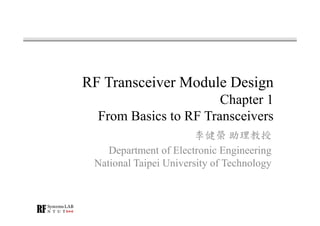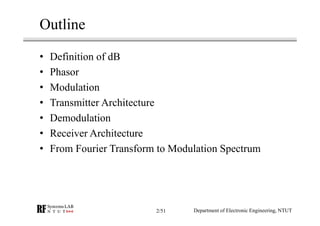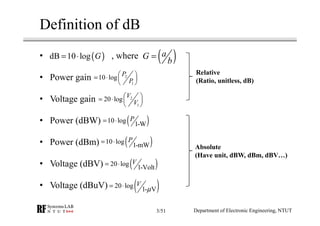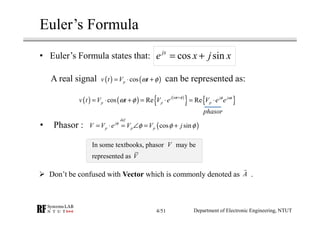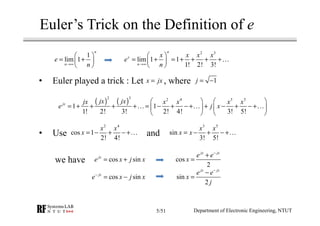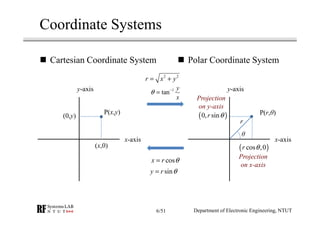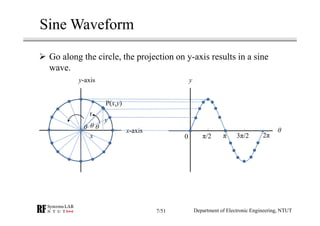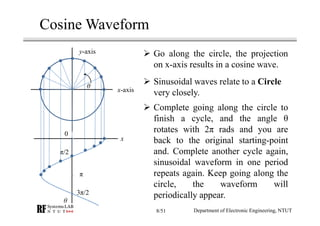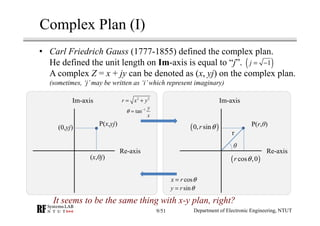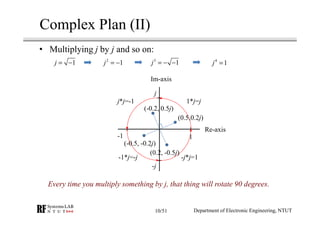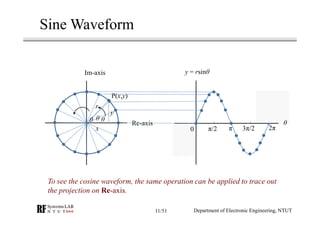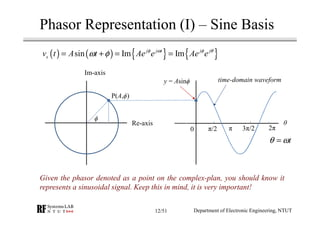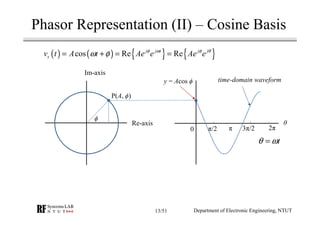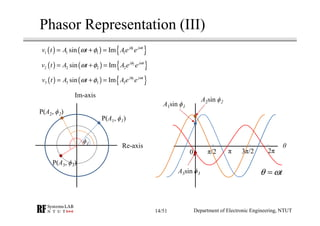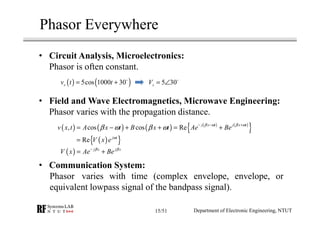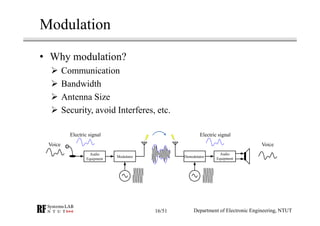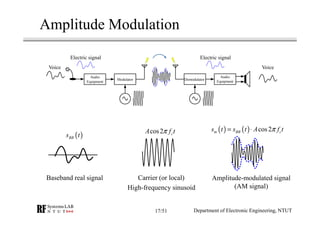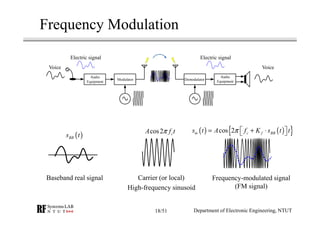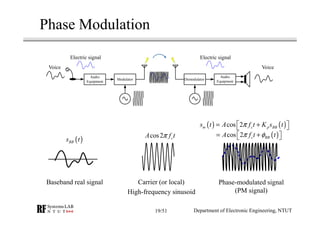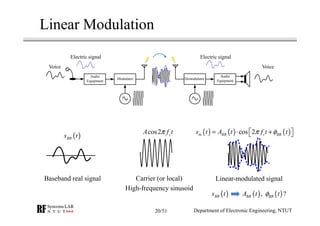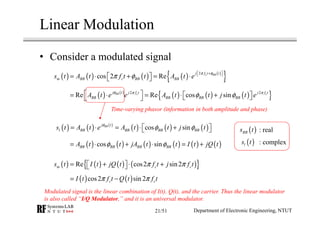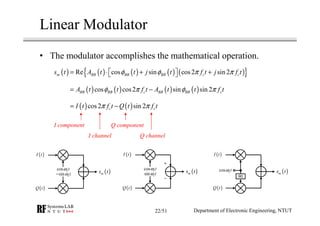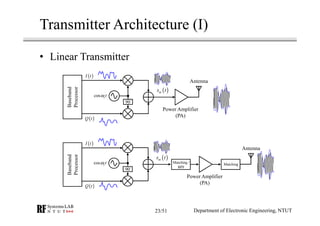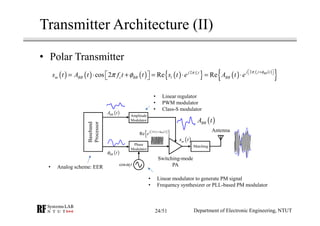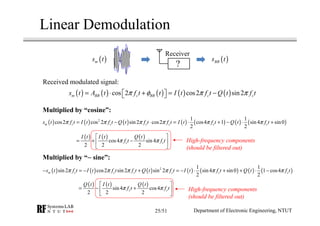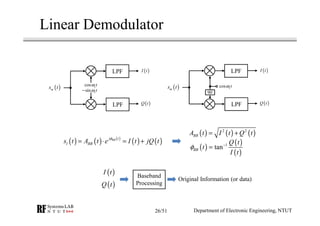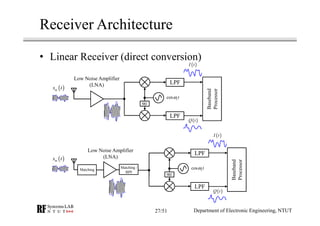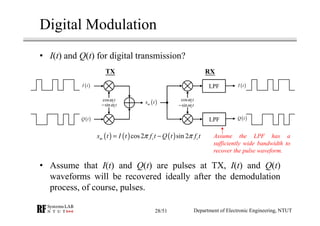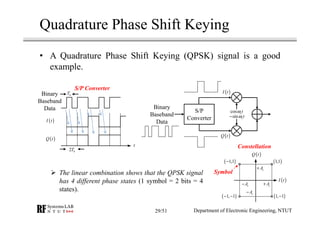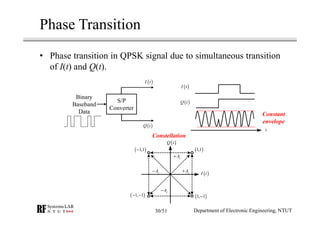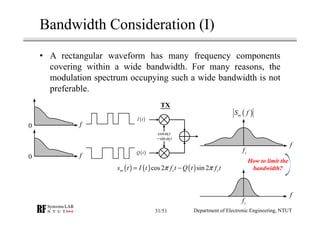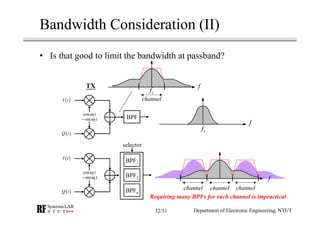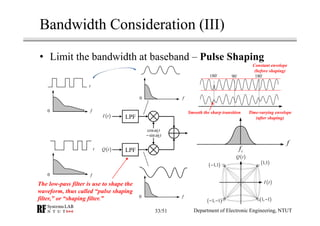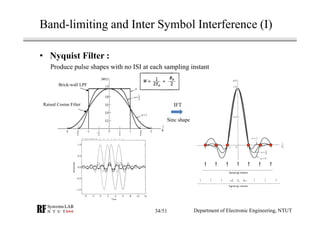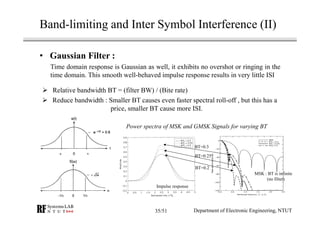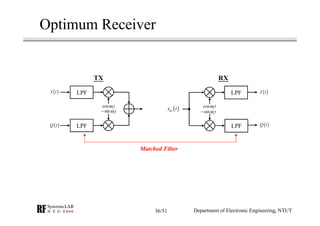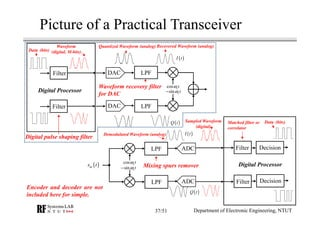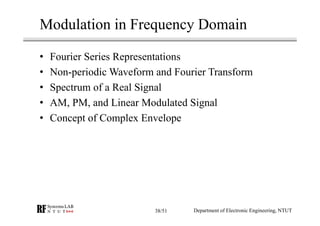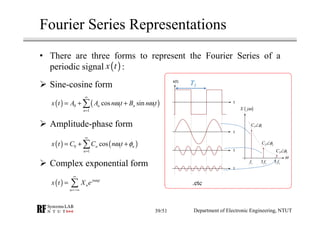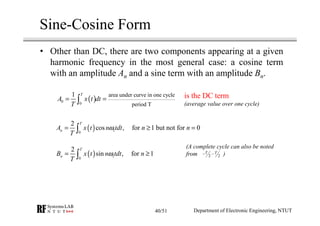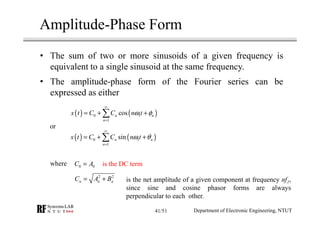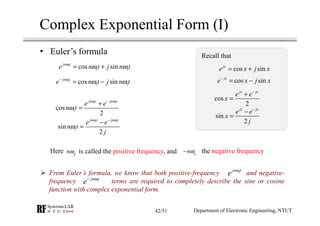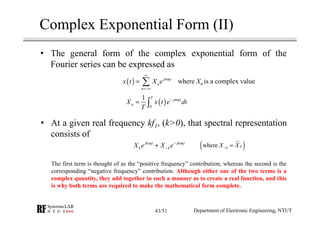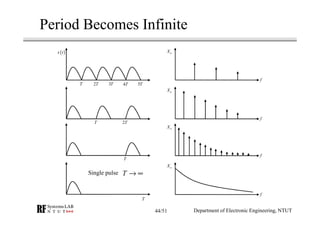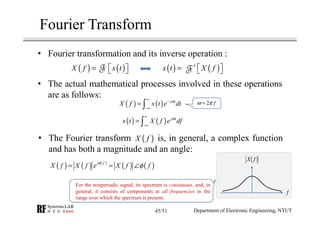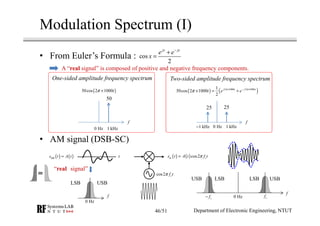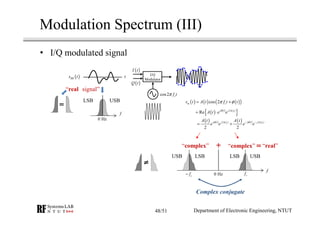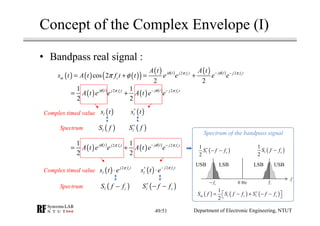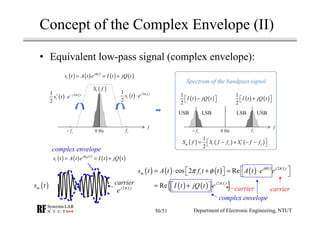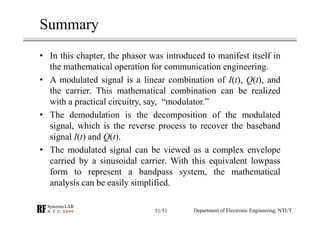1 de 51
Anúncio

### RF Module Design - [Chapter 1] From Basics to RF Transceivers

1. RF Transceiver Module Design Chapter 1 From Basics to RF Transceivers 李健榮 助理教授 Department of Electronic Engineering National Taipei University of Technology
2. Outline • Definition of dB • Phasor • Modulation • Transmitter Architecture • Demodulation • Receiver Architecture • From Fourier Transform to Modulation Spectrum Department of Electronic Engineering, NTUT2/51
3. Definition of dB • , where • Power gain • Voltage gain • Power (dBW) • Power (dBm) • Voltage (dBV) • Voltage (dBuV) ( )dB 10 log G= ⋅ ( )aG b = 2 1 10 log P P  = ⋅     2 1 20 log V V  = ⋅     ( )10 log 1-W P= ⋅ ( )10 log 1-mW P= ⋅ ( )20 log 1-Volt V= ⋅ ( )20 log 1- V V µ= ⋅ Relative (Ratio, unitless, dB) Absolute (Have unit, dBW, dBm, dBV…) Department of Electronic Engineering, NTUT3/51
4. In some textbooks, phasor may be represented as Euler’s Formula • Euler’s Formula states that: cos sinjx e x j x= + ( ) ( ) ( ) { } { }cos Re Rej t j j t p p pv t V t V e V e eω φ φ ω ω φ + = ⋅ + = ⋅ = ⋅ ( )cos sin def j p p pV V e V V jφ φ φ φ= ⋅ = ∠ = +• Phasor : Don’t be confused with Vector which is commonly denoted as .A phasor A real signal can be represented as: V V ( ) ( )cospv t V tω φ= ⋅ + Department of Electronic Engineering, NTUT4/51
5. Euler’s Trick on the Definition of e 2 3 lim 1 1 1! 2! 3! n x n x x x x e n→∞   = + = + + + +    … x jx= ( ) ( ) 2 3 2 4 3 5 1 1 1! 2! 3! 2! 4! 3! 5! jx jx jxjx x x x x e j x     = + + + + = − + − + + − + − +        … … … • Euler played a trick : Let , where 1j = − 1 lim 1 n n e n→∞   = +    6/33 2 4 cos 1 2! 4! x x x = − + − +… 3 5 sin 3! 5! x x x x= − + − +… cos sinjx e x j x= + cos sinjx e x j x− = − cos 2 jx jx e e x − + = sin 2 jx jx e e x j − − = • Use and we have Department of Electronic Engineering, NTUT5/51
6. Coordinate Systems x-axis y-axis x-axis y-axis P(r,θ) θ r P(x,y) 2 2 r x y= + 1 tan y x θ − = cosx r θ= siny r θ= Cartesian Coordinate System Polar Coordinate System (x,0) (0,y) ( )cos ,0r θ ( )0, sinr θ Projection on x-axis Projection on y-axis Department of Electronic Engineering, NTUT6/51
7. Sine Waveform x-axis y-axis P(x,y) x y r θ θθ y θ 0 π/2 π 3π/2 2π Go along the circle, the projection on y-axis results in a sine wave. Department of Electronic Engineering, NTUT7/51
8. x θ 0 π/2 π 3π/2 Cosine Waveform x-axis y-axis θ Go along the circle, the projection on x-axis results in a cosine wave. Sinusoidal waves relate to a Circle very closely. Complete going along the circle to finish a cycle, and the angle θ rotates with 2π rads and you are back to the original starting-point and. Complete another cycle again, sinusoidal waveform in one period repeats again. Keep going along the circle, the waveform will periodically appear. Department of Electronic Engineering, NTUT8/51
9. Complex Plan (I) It seems to be the same thing with x-y plan, right? • Carl Friedrich Gauss (1777-1855) defined the complex plan. He defined the unit length on Im-axis is equal to “j”. A complex Z = x + jy can be denoted as (x, yj) on the complex plan. (sometimes, ‘j’may be written as ‘i’which represent imaginary) Re-axis Im-axis Re-axis Im-axis P(r,θ) θ r P(x,yj) 2 2 r x y= + 1 tan y x θ − = cosx r θ= siny r θ= (x,0j) (0,yj) ( )cos ,0r θ ( )0, sinr θ ( )1j = − Department of Electronic Engineering, NTUT9/51
10. Complex Plan (II) Re-axis Im-axis 1 Every time you multiply something by j, that thing will rotate 90 degrees. 1j = − 2 1j = − 3 1j = − − 4 1j = 1*j=j j j*j=-1 -1 -j -1*j=-j -j*j=1 (0.5,0.2j) (-0.2, 0.5j) (-0.5, -0.2j) (0.2, -0.5j) • Multiplying j by j and so on: Department of Electronic Engineering, NTUT10/51
11. Sine Waveform Re-axis Im-axis P(x,y) x y r θ θθ y = rsinθ θ 0 π/2 π 3π/2 2π To see the cosine waveform, the same operation can be applied to trace out the projection on Re-axis. Department of Electronic Engineering, NTUT11/51
12. Phasor Representation (I) – Sine Basis ( ) ( ) { } { }sin Im Imj j t j j sv t A t Ae e Ae eφ ω φ θ ω φ= + = = Re-axis Im-axis P(A,ϕ) y = Asinϕ θ 0 π/2 π 3π/2 2π ϕ tθ ω= Given the phasor denoted as a point on the complex-plan, you should know it represents a sinusoidal signal. Keep this in mind, it is very important! time-domain waveform Department of Electronic Engineering, NTUT12/51
13. Phasor Representation (II) – Cosine Basis ( ) ( ) { } { }cos Re Rej j t j j sv t A t Ae e Ae eφ ω φ θ ω φ= + = = Re-axis Im-axis P(A, ϕ) y = Acos ϕ θ 0 π/2 π 3π/2 2π ϕ tθ ω= time-domain waveform Department of Electronic Engineering, NTUT13/51
14. Phasor Representation (III) ( ) ( ) { }1 1 1 1 1sin Im j j t v t A t Ae eφ ω ω φ= + = Re-axis Im-axis P(A1, ϕ1) ϕ1 P(A2, ϕ2) P(A3, ϕ3) θ 0 π/2 π 3π/2 2π tθ ω= A1sin ϕ1 ( ) ( ) { }2 2 2 2 2sin Im j j t v t A t A e eφ ω ω φ= + = ( ) ( ) { }3 3 3 3 3sin Im j j t v t A t A e eφ ω ω φ= + = A2sin ϕ2 A3sin ϕ3 Department of Electronic Engineering, NTUT14/51
15. Phasor Everywhere • Circuit Analysis, Microelectronics: Phasor is often constant. • Field and Wave Electromagnetics, Microwave Engineering: Phasor varies with the propagation distance. • Communication System: Phasor varies with time (complex envelope, envelope, or equivalent lowpass signal of the bandpass signal). ( ) ( )5cos 1000 30sv t t= + 5 30sV = ∠ ( ) ( ) ( ) ( ) ( ) { }, cos cos Re j x t j x t v x t A x t B x t Ae Beβ ω β ω β ω β ω − − + = − + + = + ( ) j x j x V x Ae Beβ β− = + ( ){ }Re j t V x e ω = Department of Electronic Engineering, NTUT15/51
16. Modulation • Why modulation? Communication Bandwidth Antenna Size Security, avoid Interferes, etc. Voice Electric signal Audio Equipment Audio Equipment Modulator Demodulator Electric signal Voice Department of Electronic Engineering, NTUT16/51
17. Amplitude Modulation ( ) ( ) cos2m BB cs t s t A f tπ= ⋅ Baseband real signal Voice Electric signal Audio Equipment Audio Equipment Modulator Demodulator Electric signal Voice ( )BBs t cos2 cA f tπ Carrier (or local) High-frequency sinusoid Amplitude-modulated signal (AM signal) Department of Electronic Engineering, NTUT17/51
18. Frequency Modulation ( ) ( ){ }cos 2m c f BBs t A f K s t tπ  = + ⋅  Voice Electric signal Audio Equipment Audio Equipment Modulator Demodulator Electric signal Voice Baseband real signal ( )BBs t cos2 cA f tπ Carrier (or local) High-frequency sinusoid Frequency-modulated signal (FM signal) Department of Electronic Engineering, NTUT18/51
19. Phase Modulation Voice Electric signal Audio Equipment Audio Equipment Modulator Demodulator Electric signal Voice ( ) ( )cos 2m c p BBs t A f t K s tπ = +  ( )cos 2 c BBA f t tπ φ= +   Baseband real signal ( )BBs t cos2 cA f tπ Carrier (or local) High-frequency sinusoid Phase-modulated signal (PM signal) Department of Electronic Engineering, NTUT19/51
20. Linear Modulation ( ) ( ) ( )cos 2m BB c BBs t A t f t tπ φ= ⋅ +   Voice Electric signal Audio Equipment Audio Equipment Modulator Demodulator Electric signal Voice Baseband real signal ( )BBs t cos2 cA f tπ Carrier (or local) High-frequency sinusoid Linear-modulated signal ( )BBs t ( ) ( ), ?BB BBA t tφ Department of Electronic Engineering, NTUT20/51
21. Linear Modulation • Consider a modulated signal ( ) ( ) ( ) ( ) ( ) { }2 cos 2 Re c BBj f t t m BB c BB BBs t A t f t t A t e π φ π φ +   = ⋅ + = ⋅   ( ) ( ) ( ) ( ) ( ){ }2 2 Re Re cos sinBB c cj t j f t j f t BB BB BB BBA t e e A t t j t e φ π π φ φ = ⋅ = ⋅ +    ( ) ( ) ( ) ( ) ( ) ( )cos sinBBj t l BB BB BB BBs t A t e A t t j tφ φ φ= ⋅ = ⋅ +   ( ) ( ) ( ) ( ) ( ) ( )cos sinBB BB BB BBA t t jA t t I t jQ tφ φ= ⋅ + ⋅ = + ( ) ( ) ( ) ( ){ }Re cos2 sin2m c cs t I t jQ t f t j f tπ π= + ⋅ +   ( ) ( )cos2 sin 2c cI t f t Q t f tπ π= − Time-varying phasor (information in both amplitude and phase) ( )BBs t : real ( )ls t : complex Modulated signal is the linear combination of I(t), Q(t), and the carrier. Thus the linear modulator is also called “I/Q Modulator,” and it is an universal modulator. Department of Electronic Engineering, NTUT21/51
22. Linear Modulator • The modulator accomplishes the mathematical operation. ( ) ( ) ( ) ( ) ( ){ }Re cos sin cos2 sin 2m BB BB BB c cs t A t t j t f t j f tφ φ π π= ⋅ + +   ( ) ( ) ( ) ( )cos cos2 sin sin 2BB BB c BB BB cA t t f t A t t f tφ π φ π= − ( ) ( )cos2 sin 2c cI t f t Q t f tπ π= − ( )I t cos ctω sin ctω− ( )Q t ( )ms t ( )I t cos ctω sin ctω ( )Q t ( )ms t + − 90 ( )I t cos ctω ( )Q t ( )ms t Department of Electronic Engineering, NTUT I component Q component I channel Q channel 22/51
23. Transmitter Architecture (I) • Linear Transmitter 90 ( )I t cos ctω ( )Q t ( )ms t Power Amplifier (PA) Antenna Baseband Processor 90 cos ctω ( )ms t Power Amplifier (PA) Antenna Matching / BPF Matching ( )I t ( )Q t Baseband Processor Department of Electronic Engineering, NTUT23/51
24. Transmitter Architecture (II) • Polar Transmitter ( ) ( ) ( ) ( ){ } ( ) ( ) { }22 cos 2 Re Re c BBc j f t tj f t m BB c BB l BBs t A t f t t s t e A t e π φπ π φ +   = ⋅ + = ⋅ = ⋅   ( )BBA t cos ctω ( )ms t Switching-mode PA Antenna Phase Modulator Matching ( )BBA t ( )BB tφ Baseband Processor Amplitude Modulator • Linear regulator • PWM modulator • Class-S modulator • Linear modulator to generate PM signal • Frequency synthesizer or PLL-based PM modulator • Analog scheme: EER ( ) { }2 Re c BBj f t t e π φ+   Department of Electronic Engineering, NTUT24/51
25. Linear Demodulation ( ) ( ) ( ) ( ) ( )cos 2 cos2 sin2m BB c BB c cs t A t f t t I t f t Q t f tπ φ π π= ⋅ + = −   ( ) ( ) ( ) ( ) ( ) ( ) ( )2 1 1 cos2 cos 2 sin2 cos2 cos4 1 sin4 sin0 2 2 m c c c c c cs t f t I t f t Q t f t f t I t f t Q t f tπ π π π π π= − ⋅ = ⋅ + − ⋅ + ( ) ( ) ( ) ( ) ( ) ( ) ( )2 1 1 sin2 cos2 sin2 sin 2 sin4 sin0 1 cos4 2 2 m c c c c c cs t f t I t f t f t Q t f t I t f t Q t f tπ π π π π π− = − + = − ⋅ + + ⋅ − ( ) ( ) ( )cos4 sin 4 2 2 2 c c I t I t Q t f t f tπ π   = + −    ( ) ( ) ( )sin4 cos4 2 2 2 c c Q t I t Q t f t f tπ π   = − +    ? Receiver ( )ms t ( )BBs t Received modulated signal: Multiplied by “cosine”: Multiplied by “−−−− sine”: High-frequency components (should be filtered out) High-frequency components (should be filtered out) Department of Electronic Engineering, NTUT25/51
26. Linear Demodulator ( )I t cos ctω sin ctω− ( )Q t ( )ms t LPF LPF ( )I t ( )Q t ( )ms t LPF LPF 90 cos ctω ( ) ( ) ( ) ( ) ( )BBj t l BBs t A t e I t jQ t φ = ⋅ = + ( ) ( ) ( )2 2 BBA t I t Q t= + ( ) ( ) ( ) 1 tanBB Q t t I t φ − = Baseband Processing Original Information (or data) ( )I t ( )Q t Department of Electronic Engineering, NTUT26/51
27. Receiver Architecture • Linear Receiver (direct conversion) 90 ( )I t cos ctω ( )Q t ( )ms t Low Noise Amplifier (LNA) Baseband Processor LPF LPF Matching / BPF 90 ( )I t cos ctω ( )Q t ( )ms t Low Noise Amplifier (LNA) Baseband Processor LPF LPF Matching Department of Electronic Engineering, NTUT27/51
28. Digital Modulation • I(t) and Q(t) for digital transmission? • Assume that I(t) and Q(t) are pulses at TX, I(t) and Q(t) waveforms will be recovered ideally after the demodulation process, of course, pulses. Department of Electronic Engineering, NTUT ( ) ( ) ( )cos2 sin 2m c cs t I t f t Q t f tπ π= − ( )I t cos ctω sin ctω− ( )Q t ( )ms t LPF LPF ( )I t cos ctω sin ctω− ( )Q t TX RX Assume the LPF has a sufficiently wide bandwidth to recover the pulse waveform. 28/51
29. • A Quadrature Phase Shift Keying (QPSK) signal is a good example. Quadrature Phase Shift Keying ( )I t ( )Q t ( )1,1 ( )1, 1−( )1, 1− − ( )1,1− cA+ cA+cA− cA− Constellation ( )I t cos ctω sin ctω− ( )Q t S/P Converter Binary Baseband Data( )I t ( )Q t Binary Baseband Data bT 2 bT t S/P Converter The linear combination shows that the QPSK signal has 4 different phase states (1 symbol = 2 bits = 4 states). Department of Electronic Engineering, NTUT29/51 Symbol
30. • Phase transition in QPSK signal due to simultaneous transition of I(t) and Q(t). Phase Transition ( )I t ( )Q t S/P Converter Binary Baseband Data ( )I t ( )Q t t ( )I t ( )Q t ( )1,1 ( )1, 1−( )1, 1− − ( )1,1− cA+ cA+cA− cA− Constellation Constant envelope Department of Electronic Engineering, NTUT30/51
31. Bandwidth Consideration (I) • A rectangular waveform has many frequency components covering within a wide bandwidth. For many reasons, the modulation spectrum occupying such a wide bandwidth is not preferable. ( ) ( ) ( )cos2 sin 2m c cs t I t f t Q t f tπ π= − ( )I t cos ctω sin ctω− ( )Q t TX f f f ( )mS f How to limit the bandwidth? cf cf f 0 0 Department of Electronic Engineering, NTUT31/51
32. Bandwidth Consideration (II) • Is that good to limit the bandwidth at passband? ( )I t cos ctω sin ctω− ( )Q t TX BPF cf f channel f ( )I t cos ctω sin ctω− ( )Q t BPF2 BPF1 BPFn channel f channelchannel Requiring many BPFs for each channel is impractical selector cf Department of Electronic Engineering, NTUT32/51
33. Bandwidth Consideration (III) • Limit the bandwidth at baseband – Pulse Shaping ( )I t cos ctω sin ctω− ( )Q t LPF LPF f t 0 f t 0 f0 f0 cf f The low-pass filter is use to shape the waveform, thus called “pulse shaping filter,” or “shaping filter.” Constant envelope (before shaping) 180 18090 Time-varying envelope (after shaping) Smooth the sharp transition ( )1,1 ( )1,1− ( )Q t ( )I t ( )1, 1−( )1, 1− − Department of Electronic Engineering, NTUT33/51
34. Band-limiting and Inter Symbol Interference (I) • Nyquist Filter : Produce pulse shapes with no ISI at each sampling instant Brick-wall LPF IFT Sinc shape Raised Cosine Filter Department of Electronic Engineering, NTUT34/51
35. Band-limiting and Inter Symbol Interference (II) • Gaussian Filter : Time domain response is Gaussian as well, it exhibits no overshot or ringing in the time domain. This smooth well-behaved impulse response results in very little ISI Reduce bandwidth : Smaller BT causes even faster spectral roll-off , but this has a price, smaller BT cause more ISI. Relative bandwidth BT = (filter BW) / (Bite rate) Power spectra of MSK and GMSK Signals for varying BT BT=0.2 BT=0.25 BT=0.3 Impulse response MSK : BT is infinite (no filter) Department of Electronic Engineering, NTUT35/51
36. Optimum Receiver ( )I t cos ctω sin ctω− ( )Q t ( )ms t LPF LPF ( )I t cos ctω sin ctω− ( )Q t LPF LPF TX RX Matched Filter Department of Electronic Engineering, NTUT36/51
37. Picture of a Practical Transceiver ( )I t cos ctω sin ctω− ( )Q t LPFFilter Filter DAC DAC LPF Digital Processor Digital pulse shaping filter Waveform recovery filter for DAC Data (bits) Waveform (digital, M-bits) Quantized Waveform (analog) Recovered Waveform (analog) ( )I t cos ctω sin ctω− ( )Q t ( )ms t LPF LPF Filter Filter Digital Processor Matched filter or correlator ADC ADC Demodulated Waveform (analog) Sampled Waveform (digital) Decision Decision Data (bits) Mixing spurs remover Encoder and decoder are not included here for simple. Department of Electronic Engineering, NTUT37/51
38. Modulation in Frequency Domain • Fourier Series Representations • Non-periodic Waveform and Fourier Transform • Spectrum of a Real Signal • AM, PM, and Linear Modulated Signal • Concept of Complex Envelope Department of Electronic Engineering, NTUT38/51
39. Fourier Series Representations • There are three forms to represent the Fourier Series of a periodic signal : Sine-cosine form Amplitude-phase form Complex exponential form ( ) ( )0 1 1 1 cos sinn n n x t A A n t B n tω ω ∞ = = + +∑ ( ) ( )0 1 1 cosn n n x t C C n tω φ ∞ = = + +∑ ( ) 1jn t n n x t X e ω ∞ =−∞ = ∑ ( )x t Department of Electronic Engineering, NTUT t x(t) t t t ( )X jω ω 1f 13 f 15 f .etc T1 1 1C φ∠ 2 2C φ∠ 3 3C φ∠ 39/51
40. Sine-Cosine Form ( )0 0 area under curve in one cycle period T 1 T A x t dt T = =∫ ( ) 10 2 cos , for 1 but not for 0 T nA x t n tdt n n T ω= ≥ =∫ ( ) 10 2 sin , for 1 T nB x t n tdt n T ω= ≥∫ is the DC term (average value over one cycle) • Other than DC, there are two components appearing at a given harmonic frequency in the most general case: a cosine term with an amplitude An and a sine term with an amplitude Bn. (A complete cycle can also be noted from )~ 2 2 T T− Department of Electronic Engineering, NTUT40/51
41. Amplitude-Phase Form ( ) ( )0 1 1 cosn n n x t C C n tω φ ∞ = = + +∑ ( ) ( )0 1 1 sinn n n x t C C n tω θ ∞ = = + +∑ 2 2 n n nC A B= + • The sum of two or more sinusoids of a given frequency is equivalent to a single sinusoid at the same frequency. • The amplitude-phase form of the Fourier series can be expressed as either or 0 0C A= is the DC term is the net amplitude of a given component at frequency nf1, since sine and cosine phasor forms are always perpendicular to each other. where Department of Electronic Engineering, NTUT41/51
42. Complex Exponential Form (I) 1 1 1cos sinjn t e n t j n tω ω ω= + 1 1 1cos sinjn t e n t j n tω ω ω− = − 1 1 1cos 2 jn t jn t e e n t ω ω ω − + = 1 1 1sin 2 jn t jn t e e n t j ω ω ω − − = cos sinjx e x j x= + cos sinjx e x j x− = − cos 2 jx jx e e x − + = sin 2 jx jx e e x j − − = Recall that • Euler’s formula 1 nω is called the positive frequency, and 1 nω− the negative frequency From Euler’s formula, we know that both positive-frequency and negative- frequency terms are required to completely describe the sine or cosine function with complex exponential form. Here 1jn t e ω 1jn t e ω− Department of Electronic Engineering, NTUT42/51
43. Complex Exponential Form (II) 1 1jk t jk t k kX e X eω ω− −+ ( )where kkX X− = ( ) 1jn t n n x t X e ω ∞ =−∞ = ∑ ( ) 1 0 1 T jn t nX x t e dt T ω− = ∫ • The general form of the complex exponential form of the Fourier series can be expressed as where Xn is a complex value • At a given real frequency kf1, (k>0), that spectral representation consists of The first term is thought of as the “positive frequency” contribution, whereas the second is the corresponding “negative frequency” contribution. Although either one of the two terms is a complex quantity, they add together in such a manner as to create a real function, and this is why both terms are required to make the mathematical form complete. Department of Electronic Engineering, NTUT43/51
44. Period Becomes Infinite T 2T 3T 4T 5T ( )x t f nX T 2T T T f nX f nX f nX Single pulse T → ∞ Department of Electronic Engineering, NTUT44/51
45. Fourier Transform ( ) ( )X f F x t=   F ( ) ( )1 x t F X f− =   F ( ) ( ) j t X f x t e dtω ∞ − −∞ = ∫ ( ) ( ) j t x t X f e dfω ∞ −∞ = ∫ • Fourier transformation and its inverse operation : • The actual mathematical processes involved in these operations are as follows: 2 fω π= • The Fourier transform is, in general, a complex function and has both a magnitude and an angle: ( )X f ( ) ( ) ( ) ( ) ( )j f X f X f e X f f φ φ= = ∠ ( )X f f For the nonperiodic signal, its spectrum is continuous, and, in general, it consists of components at all frequencies in the range over which the spectrum is present. Department of Electronic Engineering, NTUT45/51
46. Modulation Spectrum (I) • From Euler’s Formula : • AM signal (DSB-SC) cos 2 jx jx e e x − + = A “real signal” is composed of positive and negative frequency components. ( ) ( )cos2m cs t A t f tπ= Two-sided amplitude frequency spectrum ( ) ( )2 1000 2 10001 50cos 2 1000 2 j t j t t e eπ π π × − × × = + 2525 0 Hz 1 kHz1 kHz− f One-sided amplitude frequency spectrum 50 0 Hz 1 kHz ( )50cos 2 1000tπ × f t( ) ( )BBs t A t= f f cf0 Hzcf− 0 Hz USBLSB USBLSBLSBUSB cos2 cf tπ Department of Electronic Engineering, NTUT “real signal” 46/51
47. Phase Modulator Modulation Spectrum (II) t( )BBs t f 0 Hz USBLSB cos2 cf tπ ( ) ( )2 2 2 2 c cj t j tj f t j f tA A e e e e φ φπ π− − = + ( ) ( )( )cos 2m cs t A f t tπ φ= + ( ) { } ( ) { }2 2 Re Rec c j f t t j t j f t A e A e e π φ φ π+   = ⋅ = ⋅ Department of Electronic Engineering, NTUT “real signal” f cf0 Hzcf− USBLSBLSBUSB “complex”“complex” “real” • PM signal Complex conjugate 47/51
48. Modulation Spectrum (III) I/Q Modulator t( )BBs t f 0 Hz USBLSB cos2 cf tπ ( ) ( ) ( ) ( )2 2 2 2 c cj t j tj f t j f tA t A t e e e eφ φπ π− − = + ( ) ( ) ( )( )cos 2m cs t A t f t tπ φ= + ( ) ( ) { }2 Re cj t j f t A t e e φ π = ⋅ “real signal” • I/Q modulated signal ( )I t ( )Q t f cf0 Hzcf− USBLSBLSBUSB “complex”“complex” “real” Department of Electronic Engineering, NTUT Complex conjugate 48/51
49. Concept of the Complex Envelope (I) • Bandpass real signal : ( ) ( ) ( )( ) ( ) ( ) ( ) ( )2 2 cos 2 2 2 c cj t j tj f t j f t m c A t A t s t A t f t t e e e e φ φπ π π φ − − = + = + ( ) ( ) ( ) ( )2 21 1 2 2 c cj t j tj f t j f t A t e e A t e eφ φπ π− − = + ( )ls t ( )ls t∗ ( )lS f∗ ( )lS f Complex timed value Spectrum ( ) ( ) ( ) ( )2 21 1 2 2 c cj t j tj f t j f t A t e e A t e eφ φπ π− − = + ( ) 2 cj f t ls t e π ⋅ ( ) 2 cj f t ls t e π−∗ ⋅ ( )l cS f f∗ − −( )l cS f f− Complex timed value Spectrum ( ) ( ) ( ) 1 2 m l c l cS f S f f S f f∗  = − + − −  f cf0 Hzcf− USBLSBLSBUSB ( ) 1 2 l cS f f−( ) 1 2 l cS f f∗ − − Spectrum of the bandpass signal Department of Electronic Engineering, NTUT49/51
50. Concept of the Complex Envelope (II) • Equivalent low-pass signal (complex envelope): f 0 Hz ( )lS f cfcf− ( ) 21 2 cj f t ls t e π ⋅( ) 21 2 cj f t ls t e π−∗ ⋅ ( ) ( ) ( ) ( ) ( )j t ls t A t e I t jQ t φ = = + ( ) ( ) ( ) 1 2 m l c l cS f S f f S f f∗  = − + − −  f cf0 Hzcf− USBLSBLSBUSB ( ) ( ) 1 2 I t jQ t+   Spectrum of the bandpass signal ( ) ( ) 1 2 I t jQ t−   ( )ms t ( ) ( ) ( ) ( ) ( )BBj t ls t A t e I t jQ t φ = = + complex envelope ( ) ( ) ( ) ( ) ( ) 2 cos 2 Re cj t j f t m cs t A t f t t A t e e φ π π φ  = ⋅ + = ⋅     ( ) ( ){ }2 Re cj f t I t jQ t e π = +   complex envelope carriercarrier2 cj f t e π carrier Department of Electronic Engineering, NTUT50/51
51. Summary • In this chapter, the phasor was introduced to manifest itself in the mathematical operation for communication engineering. • A modulated signal is a linear combination of I(t), Q(t), and the carrier. This mathematical combination can be realized with a practical circuitry, say, “modulator.” • The demodulation is the decomposition of the modulated signal, which is the reverse process to recover the baseband signal I(t) and Q(t). • The modulated signal can be viewed as a complex envelope carried by a sinusoidal carrier. With this equivalent lowpass form to represent a bandpass system, the mathematical analysis can be easily simplified. Department of Electronic Engineering, NTUT51/51
Anúncio